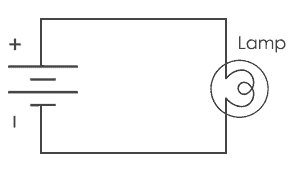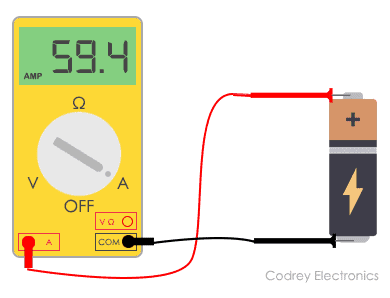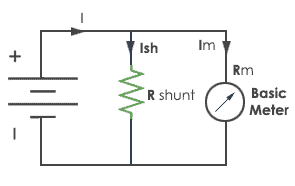# How to Measure Current in a circuit using Ammeter

Current is the measure of the rate of flow of electric charges across the conductor. It is measured in the unit of Ampere. This current measurement in a circuit is mostly done by Ammeter.

## Ammeter

Ammeter measures the electric current in the circuit. The name is derived from the SI unit of electric current, ampere. To measure electric current in a circuit, ammeter must be connected in series because, in series connection, ammeter experiences the same amount of current that flows in the circuit. Ammeter is designed to work with a small fraction of volt. So voltage drop must be minimal.

Symbol of Ammeter

The capital A represents the ammeter in the circuit.Ammeter Symbol

## How to use an Ammeter

Before we start measuring current, we will first set the ammeter range. Keeping the range to highest will prevent the ammeter to blow up its internal fuse. Then set the current type i.e. DC or AC.

Now connect the terminals of ammeter in series of the resistance or load. By this arrangement, ammeter experiences the same amount of current that flows in the circuit. For example, let us assume the simple circuit; a bulb is connected to the battery. Positive terminal of the battery is connected to positive  terminal of lamp and negative terminal of the battery is connected to negative terminal of the lamp.Now disconnect any one terminal of the bulb and connect the ammeter in such a way that one probe of ammeter is connected to the battery and another probe is connected to the bulb.

You can now observe readings in ammeter and that is the amount of current flowing in your circuit.

Now once you have noted readings from ammeter disconnect the ammeter and connect the wires as in simple circuit back.

CAUTION:

To measure current, some precautions have to be taken. Do not connect your ammeter’s probes directly to the battery to check the current of that battery. This will create a short circuit in the ammeter and sometimes this activity can blow your ammeter’s internal fuse. So please do not perform this activity.If you want to check the current of the battery. Add a resistance across the battery and connect an ammeter in series. The readings will be correct and accurate, no need to worry.Ammeter Shunt

## Other Methods to measure Current

Magnetic method

The magnetic method, we use Hall Effect to measure the current. When a wire is lying with electron flow in it, it has some current flowing inside. But they don’t have electric potential in it. If this wire is placed inside a magnetic field, difference in potential occurs perpendicular to the magnetic field and the direction of current flow.  This potential difference will be directly proportional to the current flow. Here charges interact with the magnetic field, causing the current distribution to change, which creates the Hall voltage.

The advantage of this magnetic method is that this method is capable of measuring large currents.

Measuring current using a galvanometer

A galvanometer is a device which is only used to detect the presence of current in a circuit. The deflection in the galvanometer gives the direction of flow of current i.e. if the deflection is towards the right; current is flowing in the right direction and vice versa. In galvanometer appropriate shunt resistance has been connected in parallel with the galvanometer coil, to make it into an Ammeter to measure the current.

These are two methods widely used other than measuring with an ammeter.

So, this is how you should use ammeter with all the precautions and measures. Ammeter has made calculations of current in electrical devices very easy and now with ammeter we can measure small currents in mA (milliampere) to large currents in kA (kiloampere).